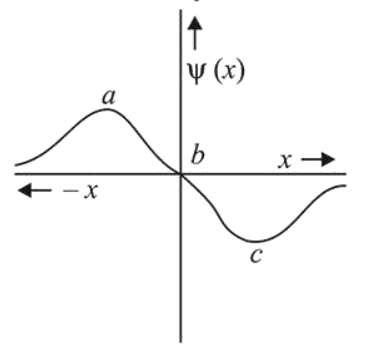# The electrons are more likely to be found:

Question:

The electrons are more likely to be found:1. in the region $a$ and $c$

2. in the region $a$ and $b$

3. only in the region $a$

4. only in the region $c$

Correct Option: 1

Solution:

Probability of finding an electron will have maximum value at both 'a' and 'c'. There is zero probability of finding an electron at ' $b$ '.